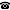# 2019 seminar talk: Residue field domination in real closed valued fields

Talk held by Clifton Ealy (Western Illinois University, Macomb, USA) at the KGRC seminar on 2019-10-03.

### Abstract

I will talk about some recent results in the model theory of valued fields. No knowledge of valued fields or model theory will be assumed.

Haskell, Hrushovski, and Macpherson show that in an algebraically closed valued field, the residue field and the value group control the rest of the structure: $tp(L/Ck(L)\Gamma(L)$ will have a unique extension to $M\supseteq C$, as long as the residue field, $k(L)$, and value group, $\Gamma(L)$, of $L$ are independent from those of $M$ (and as long as $C$ is maximal).

This behavior is striking, because it is what typically occurs in a stable structure (where types over algebraically closed sets have unique extensions to independent sets) but valued fields are far from stable, due to the order on the value group.

Real closed valued fields are even further from stable since the main sort is ordered. One might expect the analogous theorem about real closed valued fields to be that $tp(L/M)$ is implied by $tp(L/Ck(L)\Gamma(L))$ together with the order type of $L$ over $M$. In fact we show that the order type is unnecessary, that just as in the algebraically closed case, one has that $tp(L/M)$ is implied by $tp(L/k(L)\Gamma(L))$.

This is joint work with Haskell and Marikova.

A video recording of this talk is available on YouTube.

The slides are also available.

Kurt Gödel Research Center for Mathematical Logic. Währinger Straße 25, 1090 Wien, Austria.+43-1-4277-50501. Last updated: 2010-12-16, 04:37.